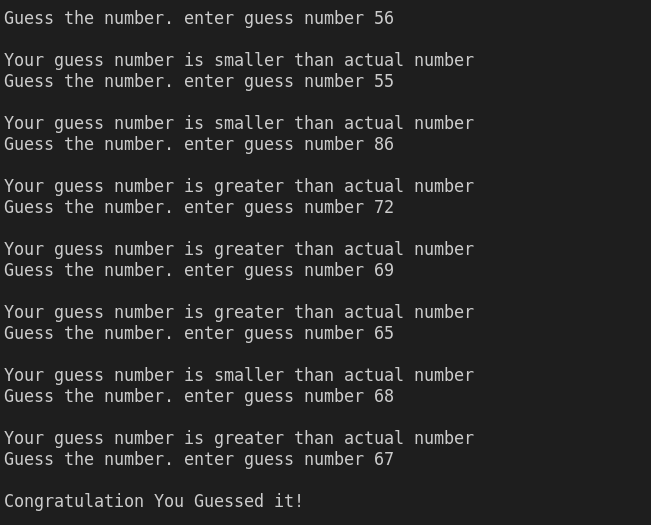Create a Guessing Game program, where you will guess a number. For now, assume any fixed number of choice as the actual number to be guessed (means, hardcode that value inside the code). Then, continuously ask the user to guess the number (I.e., input the number) unit and unless he/she guesses it correctly. Display the message(s) to the user as described below:

• If the entered number is smaller than the actual number, then you should display a message saying to the user that his/her entered number is smaller than the actual number
• If the entered number is greater than the actual number, then you should display a message saying to the user that his/her entered number is greater than the actual number
• If the user guesses the number correctly (I.e., enters the actual number), then display a Congrats message, as shown below:cpp programming exercise with solution

// #include <iostream>
// using namespace std;

// int main()
// {
// int guess_number;
// int number = 67;
// int chance = 1;
// do
// {
// cout<<"\nGuess the number. 1-100 enter guess number ";
// cin>>guess_number;
// if (guess_number >= 1 && guess_number <= 100)
// {
// if (guess_number < number)
// {
// cout<<"\nYour guess number is smaller than actual number";
// }
// else if (guess_number > number)
// {
// cout<<"\nYour guess number is greater than actual number";
// }
// else
// {
// cout<<"\nCongratulation You Guessed it!";
// }
// }
// else
// {
// cout<<"Invalid choice! :(";
// }
// if (chance > 4)
// {
// cout<<"\nSorry You Lost The Game :(";
// }
// chance++;

// } while ((chance<6) && (guess_number != number));
// return 0;
// }

#include <iostream>
using namespace std;

int main()
{
int guess_number;
int number = 67;
bool flage = true;
int count = 1;
do
{
cout<<"\nGuess the number. enter guess number ";
cin>>guess_number;
if (guess_number < number)
{
cout<<"\nYour guess number is smaller than actual number";
}
else if (guess_number > number)
{
cout<<"\nYour guess number is greater than actual number";
}
else
{
cout<<"\nCongratulation You Guessed it!";
flage = false;
}
count++;

} while (flage && count < 6);
return 0;
}

Extend the Guessing Game program created above. Other rules are the same as stated in the previous part. However, in this program, the actual number’s value should be fixed between 1 and 100 and the user should be allowed only five (05) attempts.

If the user guesses the number within five attempts, then display a Congrats message. Otherwise, the program should end after five wrong attempts, and display a sorry message to the user and the actual number as well.

#include <iostream>
using namespace std;

int main()
{
int guess_number;
int number = 67;
int chance = 1;
do
{
cout<<"\nGuess the number. 1-100 enter guess number ";
cin>>guess_number;
if (guess_number >= 1 && guess_number <= 100)
{
if (guess_number < number)
{
cout<<"\nYour guess number is smaller than actual number";
}
else if (guess_number > number)
{
cout<<"\nYour guess number is greater than actual number";
}
else
{
cout<<"\nCongratulation You Guessed it!";
}
}
else
{
cout<<"Invalid choice! :(";
}
if (chance > 4)
{
cout<<"\nSorry You Lost The Game :(";
}
chance++;

} while ((chance<6) && (guess_number != number));
return 0;
}